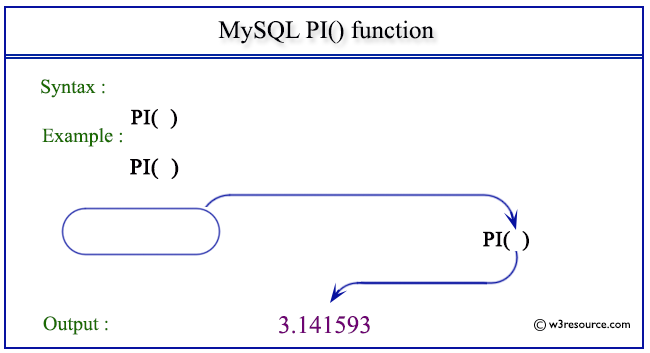# MySQL PI() function

## PI() function

MySQL PI() returns the value of π(pi).

Syntax:

```PI();
```

Pictorial presentation of MySQL PI() functionExample of MySQL PI() function

Code:

``````SELECT PI();
```
```

Explanation

The above MySQL statement returns the value of PI().

Sample Output:

```mysql> SELECT PI();
+----------+
| PI()     |
+----------+
| 3.141593 |
+----------+
1 row in set (0.00 sec)
```

All Mathematical FunctionsPrevious: OCT()
Next: POW()

﻿

Inviting useful, relevant, well-written and unique guest posts